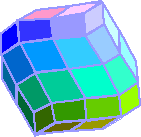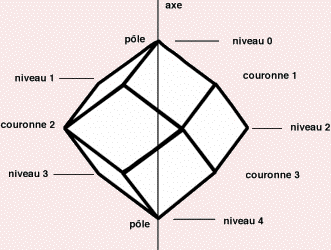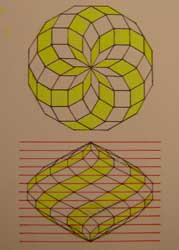un espace pour les hommesA little geometry to understand the zomePolar rhombizonahedrons of revolutionThey are volumes     diamond compounds     with 2 tops on a dummy axis (the poles)     possessing a symmetry of revolution in relation to this axis (being identical after the rotation of a certain angle around this axis)CompositionIn a crown, the rhombuses are identical. The summits are located on plans perpendicular to the axis (the levels). The distance between 2 levels is constant for a given area.3 parameters determine a precise zome        * N is the order number (integer from 3)          The crown number = N - 1 depends on it          The number of facets per crown = N          The total number of facets = N * (N - 1)Zonačdres d'ordres 4, 8 et 12* F is the number of form (integer, fractional or irrational number between 0 and infinity)The more or less pointed shape of the volume depends on itChoosing an order number and a shape number will set the shape of the volumenumber of form of 2, 1 and 0,5* A height parameter (or scale factor)If the shape is fixed, the choice of a dimension (spine or diameter or height) will determine the size of the volume and that of each of its elements